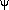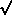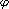# Rayleigh Scattering: Phase Function

Definitions of Angles

See Figure 11. The angle between the incident flux and the local normal is given byand µ0cos(). The angle between the local normal and the observer is given byand µcos(). The phase angle,, is the angle between the incident and emission through the origin. The azimuthal angle,, is the projection of the solar incidence and emission directions onto the local horizon. Using spherical trigonometry cosine laws (e.g., see CRC pg. 146) we can easily obtain the value of) given µ0, µ, and. For solar scattering to an observer above the atmosphere the angles are related by:

cos() =[(1-µ02)(1-µ2)] · cos() -/+ µ µ0   (S = +, T = -)

This is the method employed by Chandrasekar, Liou, and others. We could also write the equations in terms of the scattering angles. The scattering angle between incidence and emission,, which is related to the phase function, is given by=-. The scattering angle in the horizontal plane,is related to the azimuthal angle, and is given by=-. This definition is used by Hansen, Tomasko, and Danielson. It is the method employed within the VIAMP programs. Note the sign change between the two methods.

cos() =[ (1-µ02)(1-µ2)] · cos() ± µ µ0   (T = +, S = -)

The Rayleigh phase function is given in terms of scattering angles or phase angles by

Pray() = (3/4) (1 + cos2()) = (3/4)(1 + cos2()) = Pray()

The cos2 term can be expressed in terms of µ, µ0, andas

cos2() = (1-µ02)(1-µ2) · cos2() ± 2µµ0[ (1-µ02)(1-µ2) ] · cos() + (µ2 µ02)

cos(2) = 2 cos2() - 1   or,

cos2() = 1/2 cos(2) + 1/2

cos2() = 1/2 (1-µ02)(1-µ2) · cos(2) ± 2 µ µ0[ (1-µ02)(1-µ2) ] · cos() + µ2 µ02 + 1/2 (1-µ02)(1-µ2)

So the Rayleigh phase function can be written in terms of 3 Fourier moments:

Pray() = P1 + P2 cos() + P3 cos(2)

P0 = 3/4 [ 1 + µ2 µ02 + 1/2 (1-µ02)(1-µ2) ] = 3/8 ( 3 + 3 µ2 µ02022 )

P1 = ± 3/2 ( µ µ0[(1-µ02)(1-µ2)] )   (T = +, S = -)

P2 = 3/8 (1-µ02)(1-µ2)